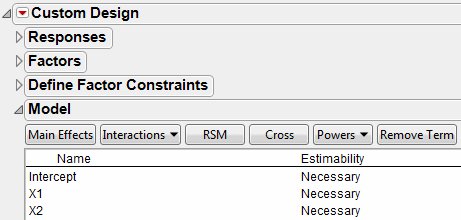Publication date: 07/30/2020

## Model

Specify your assumed model (which contains all the effects that you want to estimate) in the Model outline. For each effect that you specify, you can designate that effect’s Estimability. The Estimability value indicates whether it is Necessary to estimate that effect, or if you are content to estimate that effect If Possible.

The initial Model outline includes the main effects for all factors. If you have entered a discrete numeric factor with three or more levels, polynomial terms are also included in the initial model. The Estimability of second-and higher-order terms is set to If Possible. If you want to ensure that these terms are estimable, change their Estimability to Necessary.

Note: You can ensure that the estimability of discrete numeric polynomial terms is always set to Necessary. Select File > Preferences > Platforms > DOE. Check Discrete Numeric Powers Set to Necessary.

Figure 4.21 Model OutlineWhen you construct your design table, JMP saves a Model script to the data table. Except for discrete numeric factors, the Model script contains the effects shown in the Model outline. For a discrete numeric factor, the Model script contains only its main effect and quadratic term.

The Model outline contains the following buttons and fields:

Main Effects

Adds main effects for all factors in the model, and polynomial terms for discrete numeric factors.

Interactions

Adds interaction effects. If no factors are selected in the Factors outline, select 2nd, 3rd, 4th, or 5th to add all appropriate interactions up to that order. Add interactions up to a given order for specific factors by selecting the factor names in the Factors outline, selecting Interactions, and then specifying the appropriate order. Interactions between non-mixture and mixture factors, and interactions with blocking and constant factors, are not added.

RSM

Adds interaction and quadratic terms up to the second order (response surface model terms) for all continuous factors. Categorical factors are not included in RSM terms. Main effects for non-mixture factors that interact with all the mixture factors are removed.

Cross

Adds specific interaction terms. Select factor names in the Factors outline and effect names in the Model outline. Click Cross to add the crossed terms to the Model outline.

Powers

Adds polynomial terms. If no factor names are selected in the Factors outline, adds polynomial terms for all continuous factors. If factor names are selected in the Factors outline, adds polynomial terms for only those factors. Select 2nd, 3rd, 4th, or 5th to add polynomial terms of that order.

Scheffé Cubic

(Available for mixture factors.) Adds Scheffé cubic terms for all mixture factors. These terms are used to specify a mixture model with third-degree polynomial terms.

Remove Term

Removes selected effects.

Name

The name of the effect.

Estimability

A designation of your need to estimate the effect.

If Estimability is set to Necessary, the algorithm ensures that the effect is estimable.

If Estimability is set to If Possible, the algorithm attempts to make that effect estimable, as permitted by the number of runs that you specify.

Except for polynomial terms for discrete numeric factors, all effects are specified as Necessary by default. Click an effect’s Estimability value to change it.

#### Bayesian D-Optimality and Estimation of If Possible Effects

The Bayesian D-Optimal design approach obtains precise estimation of all Necessary terms while providing omnibus detectability (and some estimability) for If Possible terms. For more detail, see Response Surface Experiments in the Examples of Custom Designs section and Bayesian D-Optimality.